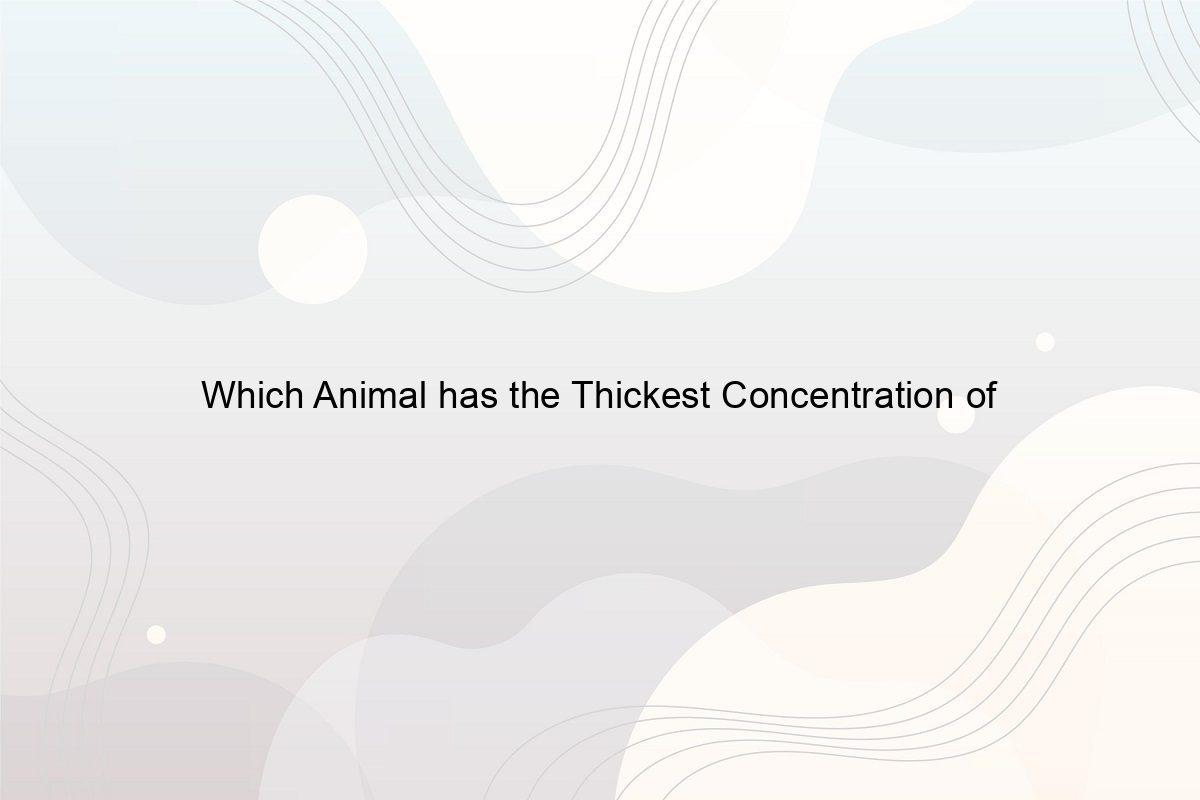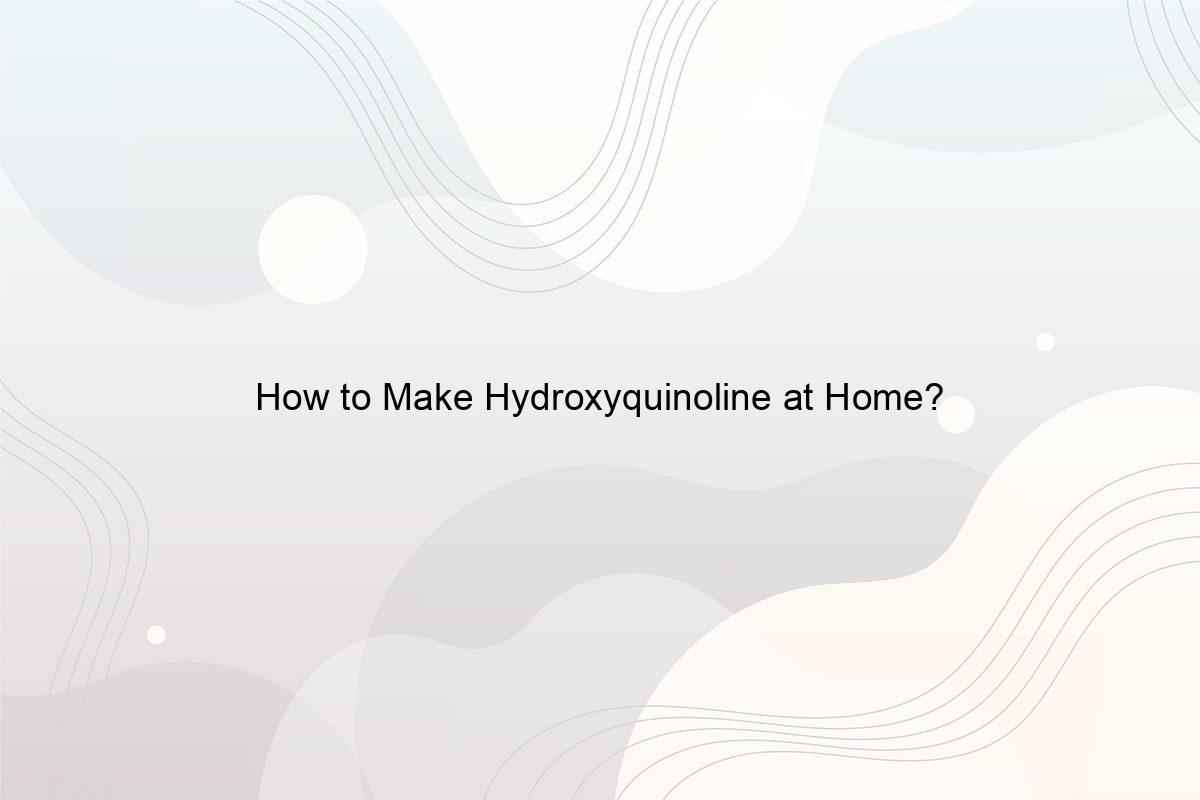﻿ 14 Sodium Facts: What is Sodium Made of? - Speeli

# 14 Sodium Facts: What is Sodium Made of?

How many Atoms does Sodium have? Does Sodium have 10 Neutrons? How many Electron Shells are in Sodium? How many Electrons are in a Sodium 22 Atom?

Do you know that you eat one of the most reactive metals every day without fail? And without this metal, you won’t be able to enjoy your food as much as you do now. Yes, you heard it right! You eat sodium metal every day through common salt. But what is sodium made of and is sodium an element? Here we will discuss every detail of sodium along with answering how many protons electrons and neutrons does sodium have and what is the formula of sodium.

### 1. Is Sodium an Element?

Sodium or Na is an element because it cannot be broken down into simpler pieces further by any chemical reaction. There are a fixed number of protons present in the element’s atom. It is a chemical element and is the sixth most commonly found element on Earth. Sodium makes up 2.6% of the Earth’s crust.  Sodium was discovered by the English chemist Humphry Davy in 1807. (See What are Examples of Elements in Everyday Life?)

### 2. What is the Mass Number of Na?

The mass number of Na is 22.989769 u which is close to 23 u. It is the eleventh element in the periodic table and hence, the atomic number is 11. It is classified as alkali metal and is solid at room temperature. It is an alkali metal that has its place in the first third period of the periodic table.

The atomic mass of any element is defined by the number of massive nuclear particles present in the atom. The mass number of an element is equal to the total number of protons plus the total number of neutrons. The total number of protons that the sodium atom has are 11 and the neutrons are 12. And when these two are added, we get the mass number of Sodium, which is 23. To know what is sodium made of, read the next segments. (See What are the Characteristics of an Element?)

### 3. What is Sodium Made of?

Photo by Jane Gonzalez on Unsplash

You already know why is sodium an element, you must have heard that sodium is one of the most common alkali metals found in abundance on Earth. It is extremely soft and can easily be cut with a knife at room temperature. It is found in the common salt which makes up the mineral halite. 80% of the dissolved constituents of the seawater are made up of sodium. Sodium is a major positive ion in fluid outside of cells. Sodium can be found in cryolite, zeolite, and sodalite. Now you know what is sodium made of. Check out what is Calcium made of?

### 4. What is the Formula of Sodium?

The formula of sodium is Na. Besides learning what is sodium made of, note that Na is the short form of the Latin word Natrium which means soda in English. Sodium is an alkali metal and has a silvery-white color and burns with a yellow flame. It reacts with water and forms hydrogen gas and sodium hydroxide. It belongs to Group 1 of the periodic table where hydrogen and alkali metals are placed. Must read how are Elements and Compounds similar and different?

### 5. How can you Write Electron Configuration for Sodium?

The electronic or electron configuration of any atom is described by the arrangement of electrons of an atom or molecule in orbitals that are present around an atomic nucleus. To know what is sodium made of internally, take a look:

• Sodium is an alkali metal that has the atomic number 11. So, the electronic configuration of the sodium (Na) element is 1s²2s²2p⁶3s¹.
• The electronic configuration for the sodium-ion (Na+) is 1s²2s²2p⁶. This is because to attain a noble gas configuration and form a sodium ion, a sodium atom has one electron in its outer shell to lose which changes its atomic or electronic configuration.

### 6. How many Atoms does Sodium have?

Sodium or Na is a chemical element. It is a highly reactive alkali metal in group 1, which contains hydrogen and alkali metals, of the periodic table. The number of atoms present in sodium is 11 and hence, the atomic number of sodium is 11. To get the atomic number of an element, we divide the weight of the respective element in grams by the AMU atomic mass given in the periodic table. The result is multiplied by Avogadro’s number. Also, check out  How many Oxygen Atoms are in SiO2?

### 7. How many Protons Electrons and Neutrons does Sodium have?

Sodium has 11 protons, 12 neutrons, and 11 electrons. We can get the answer to how many protons electrons and neutrons does sodium have using the atomic weight & atomic number of sodium. The atomic weight of sodium is 23 and the atomic number is 11.

• The atomic number of an element is always equal to the number of protons for that element. Therefore the number of protons is 11 for sodium.
• The number of neutrons can be achieved by subtracting the number of protons from the atomic weight of the element. So when we minus 11 from 23, we get 12, which is the number of neutrons of the element Sodium.
• The number of electrons is equal to the atomic number of the element. Hence, there are 11 electrons present in Sodium.

This is another way of answering what is sodium made of. (See Why do atoms form chemical bonds?)

### 8. Does Sodium have 10 Neutrons?

No, Sodium does not have 10 neutrons. You already know what is sodium made of and the fact that the number of neutrons present in sodium is 12. This is because the number of neutrons is always equal to the difference between the atomic weight and the number of protons. The number of protons in any element is always equal to the atomic number of that element. Hence, the number of protons of Sodium is 11 because the atomic number is 11. The atomic weight of sodium is 23 and when we find the difference, we get 12 as the number of neutrons. (See How does Calcium Chloride react with Chlorine?)

### 9. How many Electron Pairs can Sodium Share?

Sodium shares 1 electron to become stable. When sodium transfers or donates 1 electron, it forms an ionic bond. It gains a positive charge or you can say it becomes cations. Must see How many Electrons can a Carbon Atom share?

### 10. How many Electron Shells are in Sodium?

There are 3 electron shells present in sodium. The innermost shell contains 2 electrons. The middle shell contains 8 electrons and the outermost shell contains only 1 electron. The number of electron shells of the neutral atom can be predicted by knowing the period of an element. The horizontal row of the periodic table is called the period and all elements in the period have the same number of electron shells. Must read how many valence electrons does oxygen have?

### 11. What is the Charge of Sodium 12?

The charge of sodium or sodium 12 is +1. It is because sodium belongs to group 1. The atom of sodium has 1 electron in its outer shell. It is capable of losing one electron from its outer shell. It will have 11 positive protons and only 10 negative electrons. So, the overall charge becomes +1. The + sign is added because this atom has a positive charge and is called a cation. (See what type of ions have name ending in -ide?)

### 12. What is the Number of Neutrons of Sodium 12?

While discussing how many protons electrons and neutrons does sodium have, you have read that the number of neutrons present in sodium is 12. We can get the accurate number of neutrons by subtracting the number of protons from the atomic weight. The number of protons and electrons is always equal to the atomic number of that element. Therefore, the atomic number of sodium is 11 and the atomic weight is 23. So, when we subtract 11 from 23 (atomic weight – atomic number, which is the number of protons and electrons), we can get the number of neutrons as 12. (See What is the Number in Periodic Table of Elements H2O?)

### 13. How many Electrons are in a Sodium 22 atom?

The atomic number of sodium is 11, and the number of electrons present in Sodium 22 is 11. Sodium 22 is one of the main isotopes of sodium. It is a radioactive isotope. In this isotope, the number of protons, electrons, and neutrons is the same. All these three are 11 in number. (See What are the Components of Water?)

### 14. What is the Protons of Sodium 12?

By author Faran Raufi on Unsplash

The protons present in sodium are 11. This is because the atomic number of an element is equal to the number of protons and electrons of that particular element. The atomic number of sodium is 11, therefore, the number of protons is 11 as well.

So now you know what is sodium made of. This little element holds a great value in the environment and without it, our life becomes tasteless too. Isn’t it amazing how complex such a small thing can be? (Also read What is the pH of Concentrated Nitric Acid?)

##### Related Posts## Which Animal has the Thickest Concentration of Fur?

Why do Animals have Fur? Which Animal has the Thickest Concentration of Fur? How Dense is Dog Fur? How Dense is Cat Fur?## How to Make Hydroxyquinoline at Home?

What is Hydroxyquinoline? What are Homemade Hydroxyquinoline Benefits? Is it Safe to take Hydroxyquinoline Tablets? How to make Hydroxyquinoline with Grapefruit and Lemon?